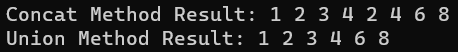# LINQ Concat Method in C#

## LINQ Concat Method in C# with Examples

In this article, I am going to discuss the LINQ Concat Method in C# with Examples. Please read our previous article before proceeding to this article where we discussed the LINQ Union Method in C# with Examples. As part of this article, we are going to discuss the following pointers.

1. What is the Concat Method in LINQ?
2. Why do we need to use the Concat Method?
3. Examples using both Query and Method Syntax.
4. What are the differences between Concat and union operators in Linq?

#### LINQ Concat Method in C#:

The LINQ Concat Method in C# is used to concatenate two sequences into one sequence. The point that you need to remember is it is used to concatenate two same types of sequences or collections and return a new sequence or collection without removing the duplicate elements. There is only one version available for this method whose signature is given below.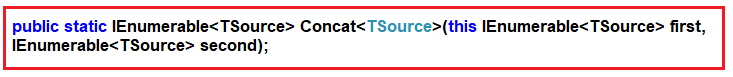##### Example to Understand Concat Method with Primitive Data Types in C#:

Let us see an example to Understand the LINQ Concat Method with Primitive Data Types in C# using both Method and Query Syntax. In the following example, we have created two integer collections which are going to be our data sources, and then we concatenate the two collections into one collection using the Concat Method. Here, you can see, both data collection data type is the same i.e. List<int>, otherwise, we will get a compilation error. In Query Syntax, there is no such operator called concat, so here we need to use the mixed syntax i.e. both the query and method syntax to achieve the same.

```using System.Linq;
using System;
using System.Collections.Generic;
namespace LINQDemo
{
class Program
{
static void Main(string[] args)
{
List<int> sequence1 = new List<int> { 1, 2, 3, 4 };
List<int> sequence2 = new List<int> { 2, 4, 6, 8 };

//Method Syntax
var MS = sequence1.Concat(sequence2);

//Query Syntax
var QS = (from num in sequence1
select num)
.Concat(sequence2).ToList();

foreach (var item in MS)
{
Console.Write(item + " ");
}

}
}
}```
###### Output: 1 2 3 4 2 4 6 8

If you notice in the above output then you will see that the duplicate elements i.e. 2 and 4 are not removed. The Concat Method will throw an exception if any of the sequences is null. In the below example, the second sequence is null and while performing the concatenate operation using the LINQ Concat Operator it will throw an exception.

```using System.Linq;
using System;
using System.Collections.Generic;
namespace LINQDemo
{
class Program
{
static void Main(string[] args)
{
List<int> sequence1 = new List<int> { 1, 2, 3, 4 };
List<int> sequence2 = null;

var result = sequence1.Concat(sequence2);

foreach (var item in result)
{
Console.WriteLine(item);
}

}
}
}```

Now run the application and you will get the following exception.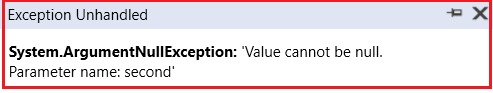##### LINQ Concat Method with Complex Data Type in C#:

Let us understand how the LINQ Concat Method works with Complex types. Create a class file with the name Student.cs and then copy and paste the following code into it.

```namespace LINQDemo
{
public class Student
{
public int ID { get; set; }
public string Name { get; set; }
}
}```

This is a very simple student class with just two properties. Let’s say, we have the following two data sources.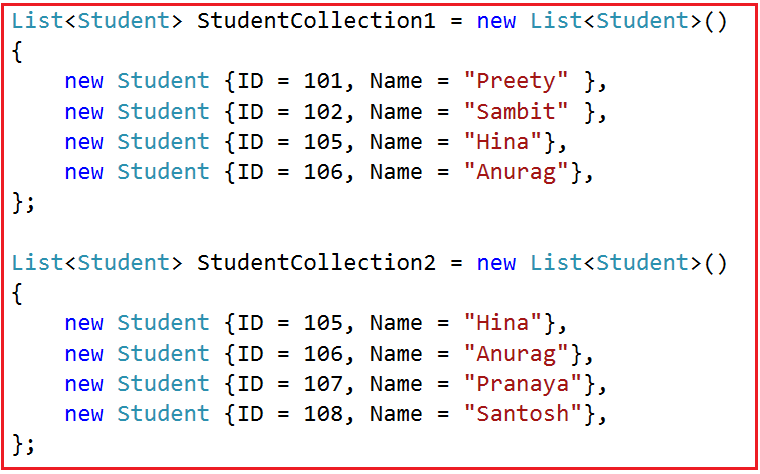Now, we need to Concatenate the above two data sources into a single data source without removing the duplicate elements. Here, you can see two students appeared in both data sources. Now, to do so, we need to use the LINQ Cancat method. So, modify the Main method of the Program class as follows.

```using System.Collections.Generic;
using System;
using System.Linq;
namespace LINQDemo
{
class Program
{
static void Main(string[] args)
{
List<Student> StudentCollection1 = new List<Student>()
{
new Student {ID = 101, Name = "Preety" },
new Student {ID = 102, Name = "Sambit" },
new Student {ID = 105, Name = "Hina"},
new Student {ID = 106, Name = "Anurag"},
};

List<Student> StudentCollection2 = new List<Student>()
{
new Student {ID = 105, Name = "Hina"},
new Student {ID = 106, Name = "Anurag"},
new Student {ID = 107, Name = "Pranaya"},
new Student {ID = 108, Name = "Santosh"},
};

//Method Syntax
var MS = StudentCollection1.Concat(StudentCollection2).ToList();

//Query Syntax
var QS = (from std in StudentCollection1
select std).Concat(StudentCollection2).ToList();

foreach (var student in MS)
{
Console.WriteLine(\$" ID : {student.ID} Name : {student.Name}");
}

}
}
}```

Now, run the application and you will get the following output which shows the duplicate elements as well.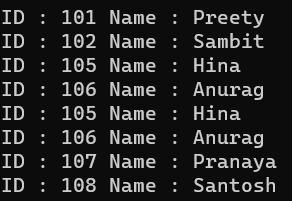Now let us concatenate the above two sequences using the Union operator and observe what happened.

##### What is the Difference Between LINQ Concat and Union Method in C#?

The Concat operator is used to concatenate two sequences into one sequence without removing the duplicate elements. That means it simply returns the elements from the first sequence followed by the elements from the second sequence. On the other hand, the LINQ Union operator is also used to concatenate two sequences into one sequence by removing duplicate elements. For a better understanding, please have a look at the following example where we are using both Cancat and Union Methods on the same data sources.

```using System.Linq;
using System;
using System.Collections.Generic;
namespace LINQDemo
{
class Program
{
static void Main(string[] args)
{
//Data Sources
List<int> sequence1 = new List<int> { 1, 2, 3, 4 };
List<int> sequence2 = new List<int> { 2, 4, 6, 8 };

//Using Concat Method
var ConcatResult = sequence1.Concat(sequence2);
Console.Write("Concat Method Result: ");
foreach (var item in ConcatResult)
{
Console.Write(item + " ");
}

//Using Union Method
var UnionResult = sequence1.Union(sequence2);
Console.Write("\nUnion Method Result: ");
foreach (var item in UnionResult)
{
Console.Write(item + " ");
}